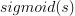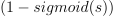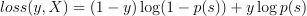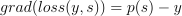## Design#

Section Full Training with OrtGradientOptimizer introduces a class able to train an ONNX graph. onnxruntime-training handles the computation of the loss, the gradient. It updates the weights as well. This design does not work when ONNX graph only plays a part in the model and is not the whole model. A deep neural network could be composed with a first layer from torch, a second layer from ONNX, and be trained by a gradient descent implemented in python.

Partial training is another way to train an ONNX model. It can be trained as a standalone ONNX graph or be integrated in a torch model or any framework implementing forward and backward mechanism. It leverages class TrainingAgent from onnxruntime-training. However a couple of lines of code are not enough to use this class. This package defines a class implementing the missing pieces: `OrtGradientForwardBackwardOptimizer`. It is initialized with an ONNX graph defining a prediction function.

```train_session = OrtGradientForwardBackwardOptimizer(
onx, ['coef', 'intercept'],
learning_rate=LearningRateSGDNesterov(),
learning_loss=ElasticLearningLoss(l1_weight=0.1, l2_weight=0.9),
learning_penalty=ElasticLearningPenalty(l1=0.1, l2=0.9))
```

The class uses onnxruntime-training to build two others, one to predict with custom weights (and not initializers), another to compute the gradient. It implements forward and backward as explained in section l-orttraining-second-api.

In addition the class holds three attributes defining the loss, its gradient, the regularization, its gradient, a learning rate possibly with momentum. They are not implemented in onnxruntime-training. That’s why they are part of this package.

Following graph summarizes how these pieces are gathered altogether. Blue piece is implemented by onnxruntime-training. Green pieces represents the three ONNX graphs needed to compute the loss and its gradient, the regularization, the weight update.The design seems over complicated compare to what pytorch does. The main reason is `torch.Tensor` supports matrix operations and class OrtValue does not. They can only be manipulated through ONNX graph and InferenceSession. These three attributes hide ONNX graph and InferenceSession to compute loss, regularization and their gradient, and to update the weights accordingly. These three classes all implement method build_onnx_function which creates the ONNX graph based on the argument the classes were initialized with. Training can then happen this way:

```train_session.fit(X_train, y_train, w_train)
```

Coefficients can be retrieved like the following:

```state_tensors = train_session.get_state()
```

And train losses:

```losses = train_session.train_losses_
```

Method `save_onnx_graph` exports all graphs used by a model. It can be saved on disk or just serialized in memory. Next examples show that in practice.

## Cache#

Base class `BaseLearningOnnx` implements methods `_bind_input_ortvalue` and `_bind_output_ortvalue` used by the three components mentioned above. They cache the binded pointers (the value returns by c_ortvalue.data_ptr() and do not bind again if the method is called again with a different OrtValue but a same pointer returned by data_ptr().

## Binary classification#

Probabilities are computed from raw scores with a function such as the sigmoid function. A binary function produces two probilities:where s is the raw score. The associated loss function is usually the log loss:where y is the expected class (0 or 1), s=s(X) is the raw score, p(s) is the probability. We could compute the gradient of the loss against the probability and let onnxruntime-training handle the computation of the gradient from the probability to the input. However, the gradient of the loss against the raw score can easily be expressed as. The second option is implemented in example Benchmark, comparison sklearn - forward-backward - classification.

## Examples#

This example assumes the loss function is not part of the graph to train but the gradient of the loss against the graph output is provided. It does not take care to the weight. This part must be separatly implemented as well. Next examples introduce how this is done with ONNX and onnxruntime-training.